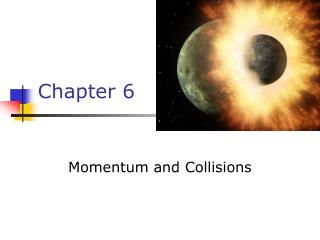DownloadDownload PresentationChapter 6

# Chapter 6

Download Presentation## Chapter 6

- - - - - - - - - - - - - - - - - - - - - - - - - - - E N D - - - - - - - - - - - - - - - - - - - - - - - - - - -
##### Presentation Transcript

1. Chapter 6 Momentum and Collisions

2. Momentum • The linear momentum of an object of mass m moving with a velocity is defined as the product of the mass and the velocity • SI Units are kg m / s • Vector quantity: the direction of the momentum is same as velocity’s

3. Momentum and Force • In order to change the momentum of an object, a force must be applied • The time rate of change of momentum of an object is equal to the net force acting on it • Gives an alternative statement of Newton’s second law

4. Impulse • When a single, constant force acts on the object, there is an impulse delivered to the object • is defined as the impulse • Vector quantity, the direction is the same as the direction of the force

5. Impulse-Momentum Theorem • The theorem states that the impulse acting on the object is equal to the change in momentum of the object • If the force is not constant, use the average force applied

6. Average Force in Impulse • The average force can be thought of as the constant force that would give the same impulse to the object in the time interval as the actual time-varying force gives in the interval

7. Impulse Applied to Auto Collisions • The most important factor is the collision time or the time it takes the person to come to a rest • This will reduce the chance of dying in a car crash • Ways to increase the time • Seat belts • Air bags Force on a car for a typical collison

8. Momentum Conservation • Mathematically: • Momentum is conserved for the system of objects • The system includes all the objects interacting with each other • Can be generalized to any number of objects

9. Example • An archer stands on frictionless ice. He fires an arrow of m = 0.500 kg with vx = 50.0 m/s. The archer and bow have mass M = 60.0 kg. • Determine the recoil speed of the archer.

10. Types of Collisions • Momentum is always conserved • Inelastic collisions • Kinetic energy is not conserved • Some kinetic energy converted to other energy such as heat, sound, work to permanently deform an object • Perfectly inelastic collisions occur when the objects stick together • Elastic collisions • both momentum and kinetic energy are conserved

11. More About Perfectly Inelastic Collisions • When two objects stick together after the collision, they have undergone a perfectly inelastic collision • Conservation of momentum becomes

12. Quick Quiz • An object of mass m moves right with speed v. It collides head-on with an object of mass 3m moving left with speed v/3. If the two stick together to have mass 4m, what is the speed of the combined object? • 0 • v/2 • v • 2v

13. Glancing Collisions • For a general collision of two objects in two-dimensional space, the conservation of momentum principle implies that the total momentum of the system in each direction is conserved • Use subscripts for identifying the object, initial and final velocities, and components

14. Glancing Collisions • The “after” velocities have x and y components • Momentum is conserved in the x direction and in the y direction • Apply conservation of momentum separately to each direction

15. Problem #42 • An 8.00 kg object moving east at 15.0 m/s on a frictionless horizontal surface collides with a 10.0 kg object at rest. After the collision, the 1st object moves South at 4.00 m/s. • Find the velocity of the 2nd object. • What percentage of the initial kinetic energy is lost in the collision?

16. Rocket Propulsion • The rocket is accelerated as a result of the thrust of the exhaust gases • This represents the inverse of an inelastic collision • Momentum is conserved • Kinetic Energy is increased (at the expense of the stored energy of the rocket fuel)

17. Rocket Propulsion • The initial mass of the rocket is M + ∆m • M is the mass of the rocket • m is the mass of the fuel • The initial velocity of the rocket is

18. More on Propulsion • The rocket’s mass is M • The mass of the fuel, ∆m, has been ejected • The rocket’s speed has increased to

19. Speed Equation • The basic equation for rocket propulsion is: • Mi is the initial mass of the rocket plus fuel • Mf is the final mass of the rocket plus any remaining fuel • The speed of the rocket is proportional to the exhaust speed

20. Thrust of a Rocket • The thrust is the force exerted on the rocket by the ejected exhaust gases • The instantaneous thrust is given by • The thrust increases as the exhaust speed increases and as the burn rate (∆M/∆t) increases